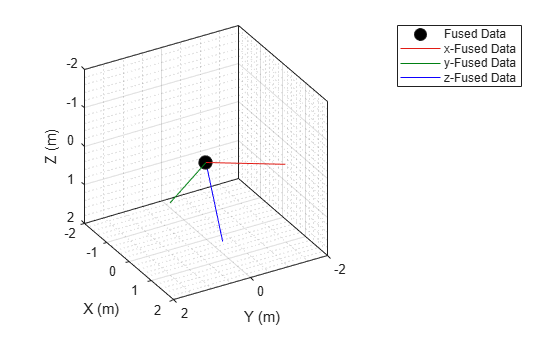# plotOrientation

Plot set of orientations in orientation plotter

## Syntax

``plotOrientation(oPlotter,orientations)``
``plotOrientation(oPlotter,roll,pitch,yaw)``
``plotOrientation(oPlotter,___,positions)``
``plotOrientation(oPlotter,___,positions,labels)``

## Description

example

````plotOrientation(oPlotter,orientations)` specifies the orientations of M objects to show for the orientation plotter, `oPlotter`. The `orientations` argument can be either an M-by-1 array of quaternions, or a 3-by-3-by-M array of rotation matrices.```
````plotOrientation(oPlotter,roll,pitch,yaw)` specifies the orientations of M objects to show for the orientation plotter, `oPlotter`. The arguments `roll`, `pitch`, and `yaw` are M-by-1 vectors measured in degrees.```
````plotOrientation(oPlotter,___,positions)` also specifies the positions of the objects as an M-by-3 matrix. Each column of `positions` corresponds to the x-, y-, and z-coordinates of the object locations, respectively.```
````plotOrientation(oPlotter,___,positions,labels)` also specifies the labels as an M-by-1 cell array of character vectors that correspond to the M orientations.```

## Examples

collapse all

This example shows how to animate the orientation of an oscillating device.

Load `rpy_9axis.mat`. The data in `rpy_9axis.mat` is recorded accelerometer, gyroscope, and magnetometer sensor data from a device oscillating in pitch (around y-axis), then yaw (around z-axis), then roll (around x-axis). The device's x-axis was pointing southward when recorded.

`ld = load('rpy_9axis.mat')`
```ld = struct with fields: Fs: 200 sensorData: [1x1 struct] ```

Set the sampling frequency. Extract the accelerometer and gyroscope data. Set the decimation factor to 2. Use `fuse` to create an indirect Kalman sensor fusion filter from the data.

```accel = ld.sensorData.Acceleration; gyro = ld.sensorData.AngularVelocity; Fs = ld.Fs; decim = 2; fuse = imufilter('SampleRate',Fs,'DecimationFactor',decim);```

Obtain the pose information of the fused data.

`pose = fuse(accel,gyro);`

Create a theater plot. Add to the theater plot an orientation plotter with `'DisplayName'` set to `'Fused Data'` and `'LocalAxesLength'` set to `2`.

```tp = theaterPlot('XLimit',[-2 2],'YLimit',[-2 2],'ZLimit',[-2 2]); op = orientationPlotter(tp,'DisplayName','Fused Data',... 'LocalAxesLength',2);```

Loop through the pose information to animate the changing orientation.

```for i=1:numel(pose) plotOrientation(op, pose(i)) drawnow end```## Input Arguments

collapse all

Orientation plotter, specified as an `orientationPlotter` object.

Orientations of M objects, specified as either an M-by-1 array of quaternions, or a 3-by-3-by-M array of rotation matrices.

Roll, pitch, and yaw angles defining the orientations of M objects, specified as M-by-1 vectors. Angles are measured in degrees.

Object positions, specified as an M-by-3 real-valued matrix, where M is the number of objects. Each column of the matrix corresponds to the x-, y-, and z-coordinates of the objects locations in meters. The default value of `positions` is at the origin.

Object labels, specified as a M-by-1 cell array of character vectors, where M is the number of objects. `labels` contains the text labels corresponding to the M objects specified in `positions`. If `labels` is omitted, no labels are plotted.